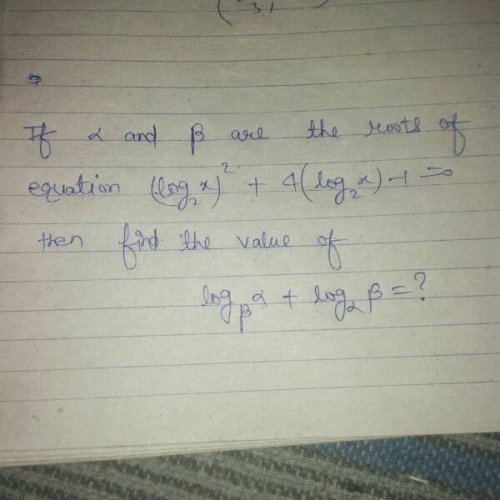JEE  >  Quadratic Equation question

# Quadratic Equation question Notes - JEE

## Document Description: Quadratic Equation question for JEE 2022 is part of JEE preparation. The notes and questions for Quadratic Equation question have been prepared according to the JEE exam syllabus. Information about Quadratic Equation question covers topics like and Quadratic Equation question Example, for JEE 2022 Exam. Find important definitions, questions, notes, meanings, examples, exercises and tests below for Quadratic Equation question.

Introduction of Quadratic Equation question in English is available as part of our JEE preparation & Quadratic Equation question in Hindi for JEE courses. Download more important topics, notes, lectures and mock test series for JEE Exam by signing up for free. JEE: Quadratic Equation question Notes - JEE
 1 Crore+ students have signed up on EduRev. Have you?please anybody solve it i have tried many times but i am not able to solve
Please this is my homework question

The document Quadratic Equation question Notes - JEE is a part of JEE category.
All you need of JEE at this link: JEE
 Use Code STAYHOME200 and get INR 200 additional OFF

Track your progress, build streaks, highlight & save important lessons and more!

,

,

,

,

,

,

,

,

,

,

,

,

,

,

,

,

,

,

,

,

,

;